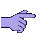## Download "Fundamentals of Mathematical Statistics " PDF Compulsory Question Paper 2021 for BCA 1st Semester Previous years Examination.

•Monday, July 25, 2022
••,
•No comments

## BCA - Bachelor of Computer Applications1st Semester ExaminationFundamentals of Mathematical Statistics

Paper: BCA-16-102

Time: 3 Hours] [Max. Marks: 65

Note:- Candidate is required to attempt five questions in all including Q. No. 9 (which is compulsory) and attempt the remaining four questions by selecting one question from each Section.

###Download "Fundamentals of Mathematical Statistics " PDF Question paper.

Section-A

1. Define Statistics. List its various functions. Discuss various types of statistical techniques. Explain the various stages involved in a statistical investigation.

2. (a) If average marks obtained by boys and girls are 68.4 and 71.2 marks respectively and average marks of whole class is 70.0 marks. Find ratio of number of boys to number of girls in class.

(b) What do you understand by Harmonic Mean? List its merits and demerits. Find harmonic mean for the following frequency distribution:

Wages in Rs. No. of Workers

40-50 12

50-60 10

60-70 15

70-80 17

80-90 8

90-100 3

Section-B

3. (a) What do you mean by Partition Values ? In what respect they are different from median? Explain any three partition values with their formulas.

(b) The median of the following data is 20.75. Find the missing frequencies x and y if total frequencies is 100:

Class Interval Frequency

0-5 7

5-10 10

10-15 x

15-20 13

20-25 y

25–30 10

30-35 14

35-40 9

4. (a) The mean and standard deviation of 100 items were calculated as 50 and 6 respectively. Later on, it was discovered that a value 65 misread as 56. Find out correct mean and standard deviation.

(b)Find out mode for the following data:

Marks No. of Students

10-19 10

20-29 12

30-39 18

40-49 30

50-59 16

60-69 6

70–79 8

Section-C

5. (a) Calculate Karl Pearson coefficient of correlation and interpret the result, between the values of X and Y given below. Take A = Assumed mean from X series = 69; B = Assumed mean from Y series = 112.

(b) Rank correlation of marks obtained by 10 students was found to 0.2. It was later discovered that difference in ranks in two items was wrongly taken as 9 instead of 7. Find the correct value of coefficient of rank correlation.

6. (a) What is a Scatter Diagram ? How does it help us in studying the correlation between two variables, in respect of both nature and extent?

(b) What are the advantages of Spearman's rank no correlation over Karl Pearson correlation coefficient? Calculate rank correlation from the following scores:

Section-D

7. What is Regression Analysis? What is the difference between correlation and regression analysis? Explain the method of least square to fit a straight line which is the best fit.

8. From the following data, obtain the two regression equation:

Section-E

(Compulsory Question)

9. (a) Define interquartile range.

(b) Define Bivariate frequency distribution.

(c) What is the relationship between standard deviation and coefficient of variation?

(d) Mention any two limitations of regression analysis.

(e) Define mean deviation.

(f) The mean and mode in a moderately symmetrical distribution are 24.6 and 26.1 respectively. Find the median.